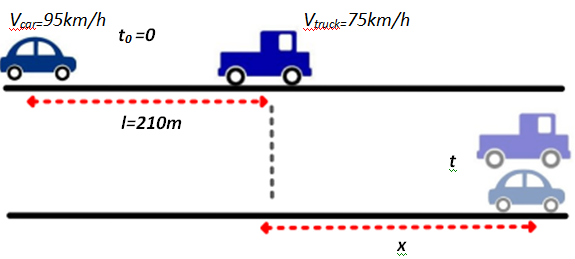×
Get Full Access to Physics: Principles With Applications - 6 Edition - Chapter 2 - Problem 12p
Get Full Access to Physics: Principles With Applications - 6 Edition - Chapter 2 - Problem 12p

×

# (II) A car traveling 95 km/h is 210 m behind a truckISBN: 9780130606204 3

## Solution for problem 12P Chapter 2

Physics: Principles with Applications | 6th Edition

• Textbook Solutions
• 2901 Step-by-step solutions solved by professors and subject experts
• Get 24/7 help from StudySoup virtual teaching assistantsPhysics: Principles with Applications | 6th Edition

4 5 1 277 Reviews
19
2
Problem 12P

Problem 12P

(II) A car traveling 95 km/h is 210 m behind a truck traveling 75 km /How long will it take the car to reach the truck?

Step-by-Step Solution:

Step 1 of 4At the beginning, the car is 210m behind the car and at a speed of 95 km/h. The truck is moving at a speed of 75km/h. Since the car is faster at time t the car catches the truck. We will  the time when the car reaches the truck.

Step 2 of 4

Step 3 of 4

##### ISBN: 9780130606204

The answer to “(II) A car traveling 95 km/h is 210 m behind a truck traveling 75 km /How long will it take the car to reach the truck?” is broken down into a number of easy to follow steps, and 26 words. Since the solution to 12P from 2 chapter was answered, more than 1182 students have viewed the full step-by-step answer. This full solution covers the following key subjects: TRUCK, Car, traveling, reach, take. This expansive textbook survival guide covers 35 chapters, and 3914 solutions. Physics: Principles with Applications was written by and is associated to the ISBN: 9780130606204. This textbook survival guide was created for the textbook: Physics: Principles with Applications, edition: 6. The full step-by-step solution to problem: 12P from chapter: 2 was answered by , our top Physics solution expert on 03/03/17, 03:53PM.

## Discover and learn what students are asking

Calculus: Early Transcendental Functions : Space Coordinates and Vectors in Space
?In Exercises 1-4, plot the points in the same three-dimensional coordinate system. (a) (3, -2, 5)

Statistics: Informed Decisions Using Data : Introduction to the Practice of Statistics
?____________ are the characteristics of the individuals of the population being studied

Statistics: Informed Decisions Using Data : Testing the Significance of the Least-Squares Regression Model
?The output shown was obtained from Minitab. (a) The least-squares regression equation is yn = 1.3962x + 12.396. What is the predict

Chemistry: The Central Science : The Chemistry of Life: Organic and Biological Chemistry
?Give the the name or condensed structural formula, as appropriate: (c) 2-me

Unlock Textbook Solution

Enter your email below to unlock your verified solution to:

(II) A car traveling 95 km/h is 210 m behind a truck